# 图解冒泡排序，选择排序，插入排序与希尔排序⭐️前面的话⭐️

📒博客主页：未见花闻的博客主页
🎉欢迎关注🔎点赞👍收藏⭐️留言📝
📌本文由未见花闻原创！
📆掘金首发时间：🌴2022年4月28日🌴
✉️坚持和努力一定能换来诗与远方！
💭参考书籍：📚《java编程思想》，📚《java核心技术》，📚《数据结构》
💬参考在线编程网站：🌐牛客网🌐力扣

🍭作者水平很有限，如果发现错误，一定要及时告知作者哦！感谢感谢！

# 2.冒泡排序

## 2.1排序算法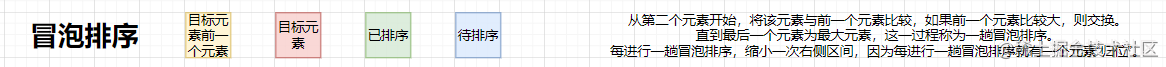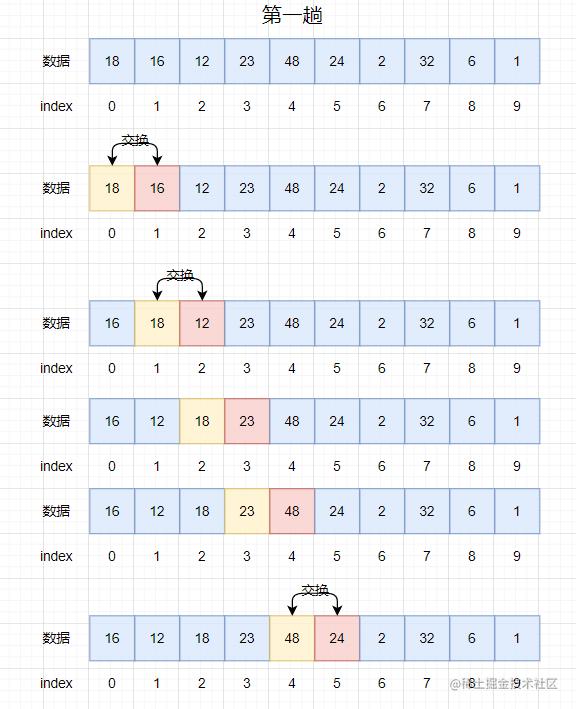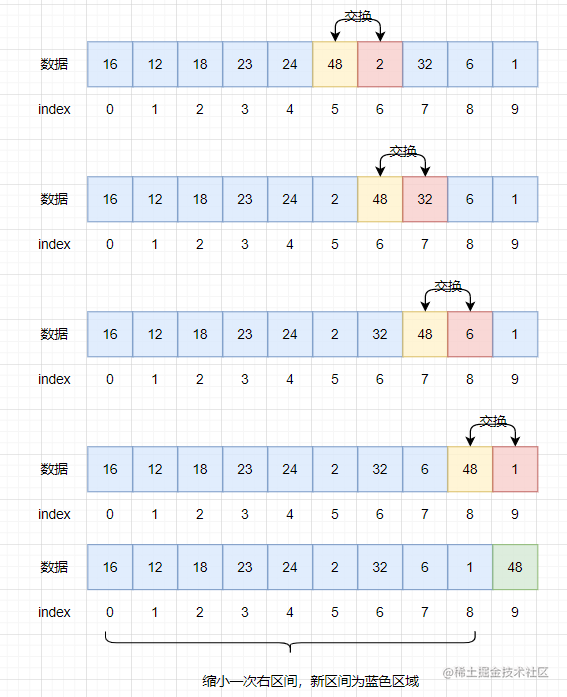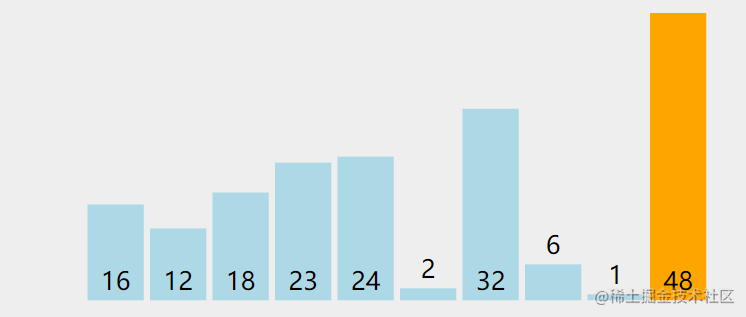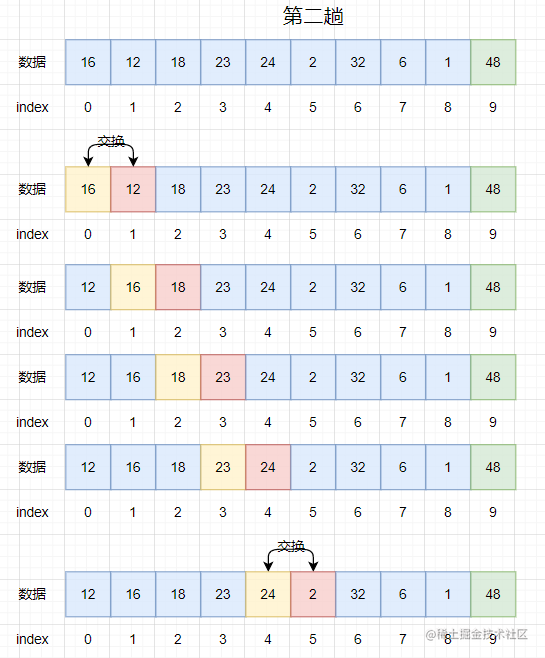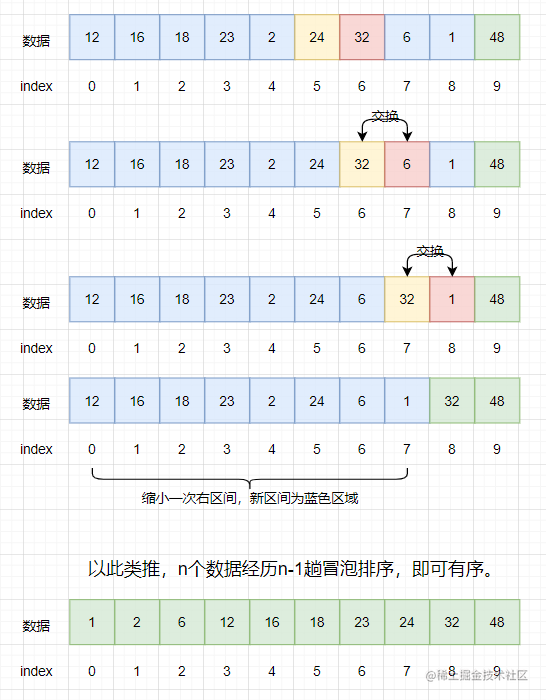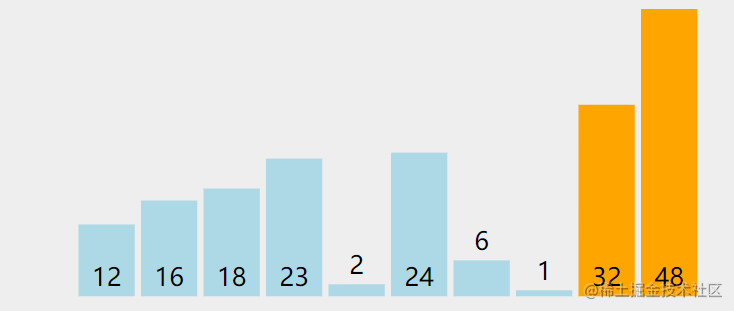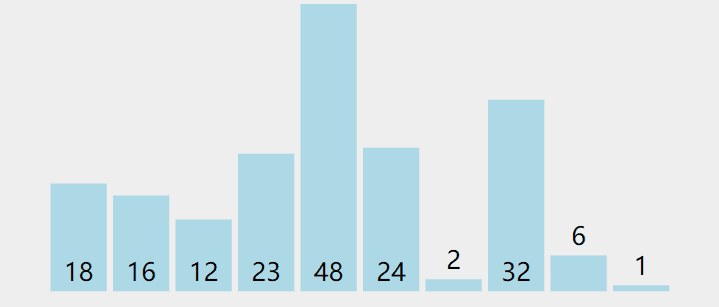自定义交换数组元素方法：

    public void swap(int[] arr, int index1, int index2) {
int tmp = arr[index1];
arr[index1] = arr[index2];
arr[index2] = tmp;
}

    /**
* 冒泡排序
* @param array 排序对象
*/
//优化前
public void bubbleSort(int[] array) {
for (int i = 0; i < array.length - 1; i++) {    //排序趟数
for (int j = 1; j < array.length - i; j++) {
//如果j所有处元素小于j-1索引处元素，则交换，否则不交换
if (array[j] < array[j - 1]) {
swap(array, j, j-1);
}
}
}
}

    //优化后
public void bubbleSort(int[] array) {
for (int i = 0; i < array.length - 1; i++) {    //排序趟数
boolean flag = false;                       //标记
for (int j = 1; j < array.length - i; j++) {
//如果j所有处元素小于j-1索引处元素，则交换，否则不交换
if (array[j] < array[j - 1]) {
swap(array, j, j-1);
flag = true;                        //发生交换将标记置为真
}
}
if (!flag) break;                           //一趟冒泡排序后flag为false说明数组已经有序了
}
}

## 2.2性能分析

$O(N^2)$$O(1)$相同值元素相对位置不变，稳定

# 3.选择排序

## 3.1排序算法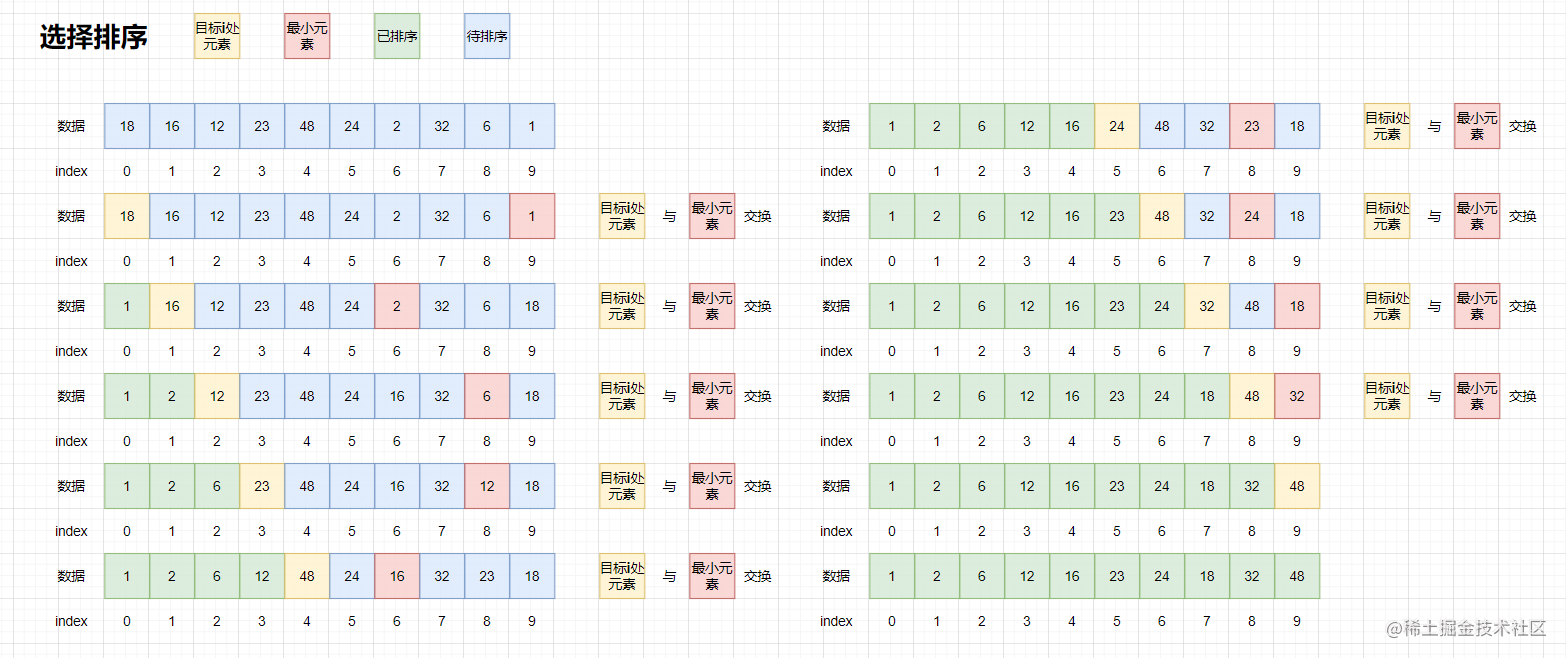/**
* 选择排序
* @param array 待排序序列
*/
public void selectSort(int[] array) {
int minIndex = 0;                                    //存放最小元素的下标
for (int i = 0; i < array.length - 1; i++) {
minIndex = i;                                   //默认为i下标
for (int j = i + 1; j < array.length; j++) {
if (array[j] < array[minIndex]) {
minIndex = j;                           //获取i下标后面更小元素的下标
}
}
swap(array, i, minIndex);                       //交换
}
}

## 3.2性能分析

$O(N^2)$$O(1)$跳跃位置交换，可能存在相同值元素相对位置改变，不稳定

# 4.插入排序

## 4.1直接插入排序

### 4.1.1排序算法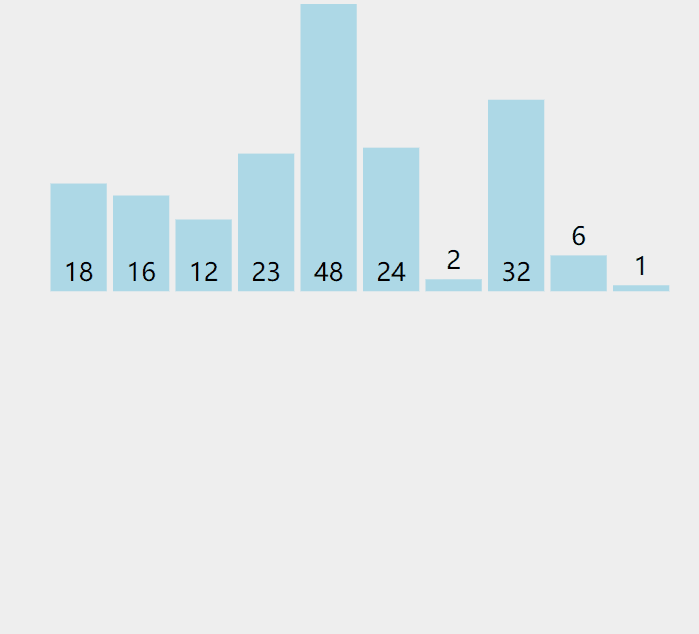/**
* 直接插入排序
* @param array 待排序对象
*/
public void insertSort(int[] array) {
for (int i = 1; i < array.length; i++) {
int insert = array[i];                      //待插入元素
int index = i - 1;                          //待插入元素前一个元素下标
while (index >= 0) {
if (array[index] > insert) {
array[index + 1] = array[index];          //在数组下标合法范围内，比较insert与index位置元素大小，如果index位置元素更大则将此元素放入index+1位置，index--
index--;
}else {
break;                                  //否则结束插入
}
}
array[index+1] = insert;                          //将insert插入index+1位置处
}
}

### 4.1.2性能分析

$O(N^2)$$O(1)$相同数字排序相对顺序不改变，稳定

## 4.2希尔排序

### 4.2.1排序算法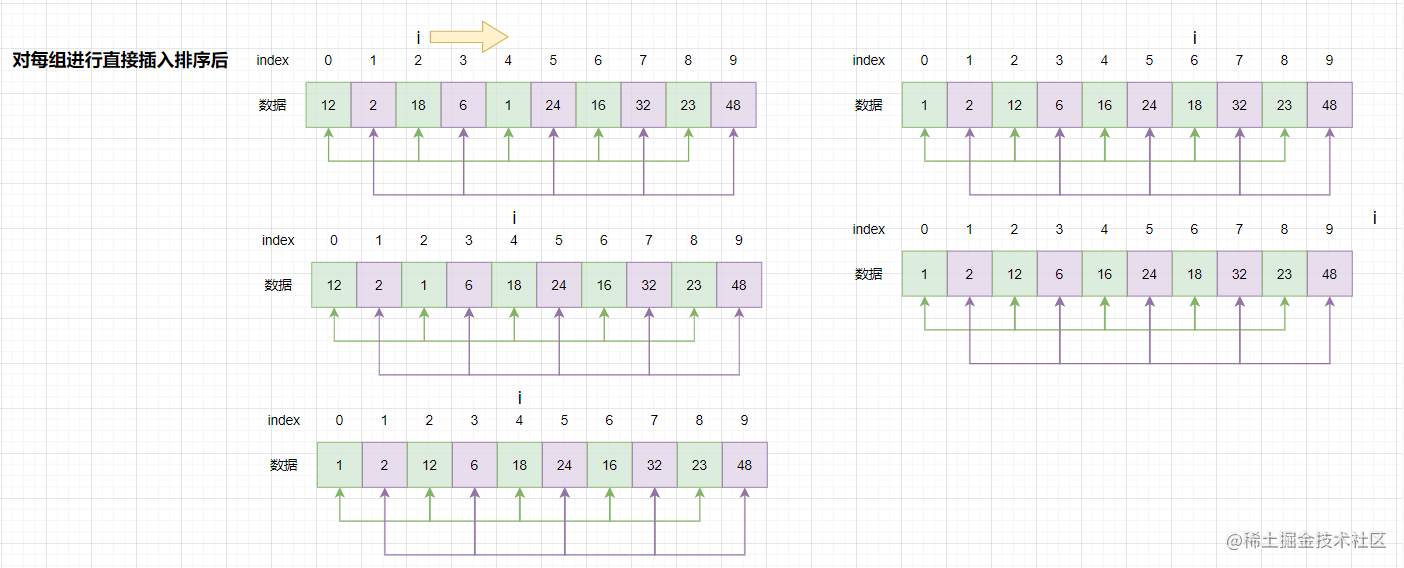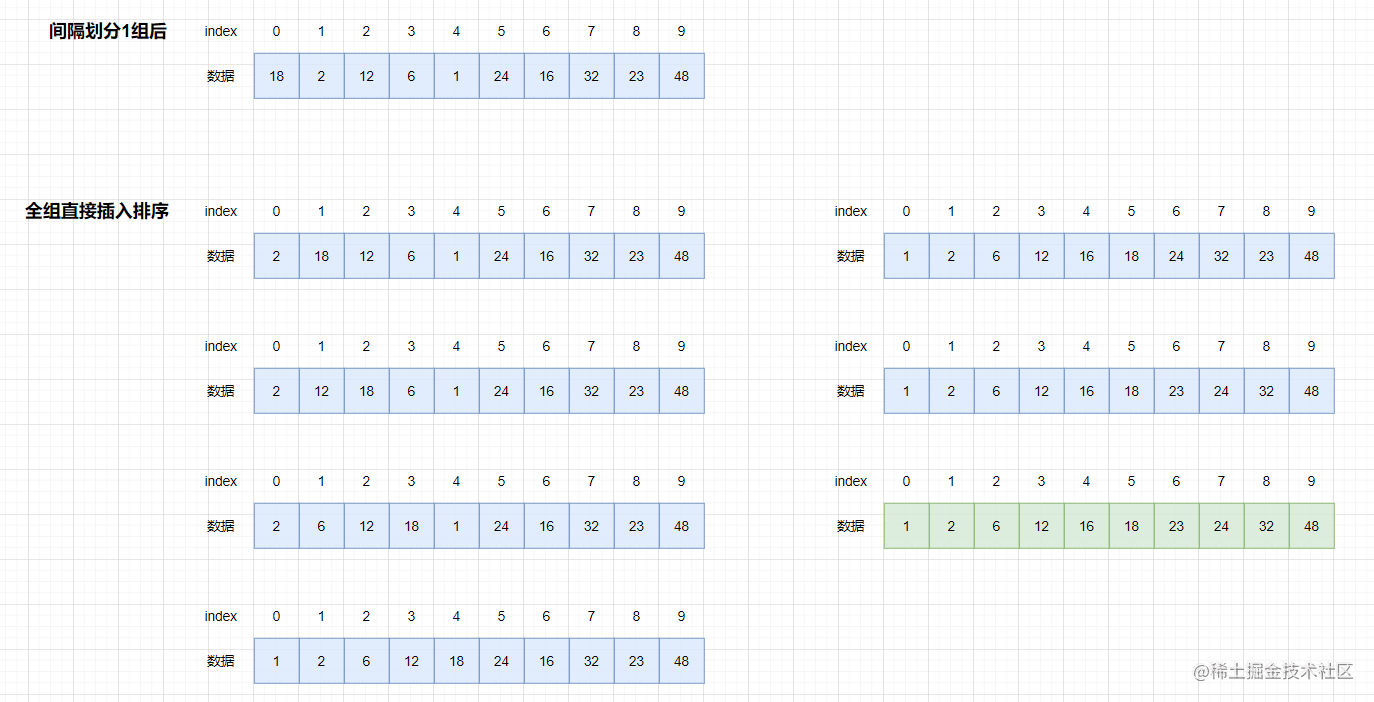/**
* 希尔排序
* @param array 待排序序列
* @param gap 组数
*/
public void shell(int[] array,int gap) {
int insert = 0;
for (int i = gap; i < array.length; i++) {
insert = array[i];
int j = i - gap;
while (j >= 0) {
if (array[j] > insert) {
array[j + gap] = array[j];
j -= gap;
} else {
break;
}
}
array[j + gap] = insert;
}
}

public void shellSort(int[] array) {
int gap = array.length;
while (gap > 1) {
shell(array,gap);
gap /= 2;
}
shell(array,1);//保证最后是1组
}

### 4.2.2性能分析

$O(N^{1.3} )-O(N^{1.5})$$O(1)$不稳定